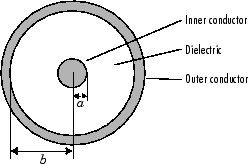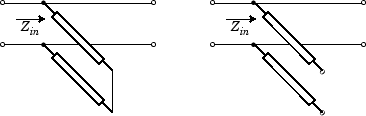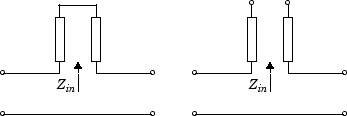# rfckt.coaxial

Coaxial transmission line

## Description

Use the `rfckt.coaxial` object to create a coaxial transmission line that is characterized by line dimensions, stub type, and termination.

A coaxial transmission line is shown in cross-section in the following figure. Its physical characteristics include the radius of the inner conductor of the coaxial transmission line a, and the radius of the outer conductor b.Note

`txlineCoaxial` is recommended over `rfckt.coaxial` because it enables you to:

(since R2023b)

## Creation

### Syntax

``h = rfckt.coaxial``
``h = rfckt.coaxial(Name,Value)``

### Description

example

````h = rfckt.coaxial` returns a coaxial transmission line object whose properties are set to their default values.```
````h = rfckt.coaxial(Name,Value)` sets properties using one or more name-value pairs. For example, `rfckt.coaxial('OuterRadius',0.0043)` creates a coaxial transmission line object with outer radius of 0.0043 meters. You can specify multiple name-value pairs. Enclose each property name in quotes. Properties not specified retain their default values.```

## Properties

expand all

Computed S-parameters, noise figure, OIP3, and group delay values, specified as an `rfdata.data` object. This is a read-only property. For more information refer, Algorithms.

Data Types: `function_handle`

Relative permittivity of dielectric, specified as a scalar. The relative permittivity is the ratio of permittivity of the dielectric,$\epsilon$, to the permittivity in free space, ${\epsilon }_{0}$. The default value is`2.3`.

Data Types: `double`

Inner conductor radius, specified as a scalar in meters. The default value is `7.25e-4`.

Data Types: `double`

Physical length of transmission line, specified as a scalar in meters. The default value is `0.01`.

Data Types: `double`

Tangent of loss angle of dielectric, specified as a scalar. The default value is `0`.

Data Types: `double`

Relative permeability of dielectric, specified as a scalar. The ratio of permeability of dielectric, $\mu$, to the permeability in free space, ${\mu }_{0}$. The default value is `1`.

Data Types: `double`

Object name, specified as a `1-by-N` character array.

Data Types: `char`

Number of ports, specified as a positive integer.

Data Types: `double`

Outer conductor radius, specified as a scalar in meters. The default value is `0.0026`.

Data Types: `double`

Conductor conductivity, specified as a scalar in Siemens per meter (S/m). The default value is `Inf`.

Data Types: `double`

Type of stub, specified as one of the following values: `'NotaStub'`, `'Series'`, `'Shunt'`.

Data Types: `double`

Stub transmission line termination, specified as one of the following values: `'NotApplicable'`, `'Open'`, `'Short'`.

Data Types: `double`

## Object Functions

 `analyze` Analyze RFCKT object in frequency domain `calculate` Calculate specified parameters for rfckt objects or rfdata objects `circle` Draw circles on Smith Chart `extract` Extract specified network parameters from rfckt object or data object `listformat` List valid formats for specified circuit object parameter `listparam` List valid parameters for specified circuit object `loglog` Plot specified circuit object parameters using log-log scale `plot` Plot circuit object parameters on X-Y plane `plotyy` Plot parameters of RF circuit or RF data on X-Y plane with two Y-axes `getop` Display operating conditions `polar` Plot specified object parameters on polar coordinates `semilogx` Plot RF circuit object parameters using log scale for x-axis `semilogy` Plot RF circuit object parameters using log scale for y-axis `smith` Plot circuit object parameters on Smith chart `write` Write RF data from circuit or data object to file `getz0` Calculate characteristic impedance of RFCKT transmission line object `read` Read RF data from file to new or existing circuit or data object `restore` Restore data to original frequencies `getop` Display operating conditions

## Examples

collapse all

Create a coaxial transmission line with 0.0045 meters outer radius using rfckt.coaxial.

`tx1=rfckt.coaxial('OuterRadius',0.0045)`
```tx1 = rfckt.coaxial with properties: OuterRadius: 0.0045 InnerRadius: 7.2500e-04 MuR: 1 EpsilonR: 2.3000 LossTangent: 0 SigmaCond: Inf LineLength: 0.0100 StubMode: 'NotAStub' Termination: 'NotApplicable' nPort: 2 AnalyzedResult: [] Name: 'Coaxial Transmission Line' ```

## Algorithms

The `analyze` method treats the transmission line as a 2-port linear network. It computes the `AnalyzedResult` property of a stub or as a stubless line using the data stored in the `rfckt.coaxial` object properties as follows:

• If you model the transmission line as a stubless line, the `analyze` method first calculates the ABCD-parameters at each frequency contained in the modeling frequencies vector. It then uses the `abcd2s` function to convert the ABCD-parameters to S-parameters.

The `analyze` method calculates the ABCD-parameters using the physical length of the transmission line, d, and the complex propagation constant, k, using the following equations:

`$\begin{array}{l}A=\frac{{e}^{kd}+{e}^{-kd}}{2}\\ B=\frac{{Z}_{0}*\left({e}^{kd}-{e}^{-kd}\right)}{2}\\ C=\frac{{e}^{kd}-{e}^{-kd}}{2*{Z}_{0}}\\ D=\frac{{e}^{kd}+{e}^{-kd}}{2}\end{array}$`

Z0 and k are vectors whose elements correspond to the elements of f, the vector of frequencies specified in the `analyze` input argument `freq`. Both can be expressed in terms of the resistance (R), inductance (L), conductance (G), and capacitance (C) per unit length (meters) as follows:

`$\begin{array}{c}{Z}_{0}=\sqrt{\frac{R+j2\pi fL}{G+j2\pi fC}}\\ k={k}_{r}+j{k}_{i}=\sqrt{\left(R+j2\pi fL\right)\left(G+j2\pi FC\right)}\end{array}$`

where

`$\begin{array}{c}R=\frac{1}{2\pi {\sigma }_{cond}{\delta }_{cond}}\left(\frac{1}{a}+\frac{1}{b}\right)\\ L=\frac{\mu }{2\pi }\mathrm{ln}\left(\frac{b}{a}\right)\\ G=\frac{2\pi \omega {\epsilon }^{″}}{\mathrm{ln}\left(\frac{b}{a}\right)}\\ C=\frac{2\pi \epsilon }{\mathrm{ln}\left(\frac{b}{a}\right)}\end{array}$`

In these equations:

• a is the radius of the inner conductor.

• b is the radius of the outer conductor.

• σcond is the conductivity in the conductor.

• μ is the permeability of the dielectric.

• ε is the permittivity of the dielectric.

• ε″ is the imaginary part of ε, ε″  = ε0εrtan δ, where:

• ε0 is the permittivity of free space.

• εr is the `EpsilonR` property value.

• tan δ is the `LossTangent` property value.

• δcond is the skin depth of the conductor, which the method calculates as $1/\sqrt{\pi f\mu {\sigma }_{cond}}$.

• f is a vector of modeling frequencies determined by the Outport (RF Blockset) block.

• If you model the transmission line as a shunt or series stub, the `analyze` method first calculates the ABCD-parameters at the specified frequencies. It then uses the `abcd2s` function to convert the ABCD-parameters to S-parameters.

When you set the `StubMode` property to `'Shunt'`, the 2-port network consists of a stub transmission line that you can terminate with either a short circuit or an open circuit as shown in the following figure.Zin is the input impedance of the shunt circuit. The ABCD-parameters for the shunt stub are calculated as:

`$\begin{array}{c}A=1\\ B=0\\ C=1/{Z}_{in}\\ D=1\end{array}$`

When you set the `StubMode` property to `'Series'`, the 2-port network consists of a series transmission line that you can terminate with either a short circuit or an open circuit as shown in the following figure.Zin is the input impedance of the series circuit. The ABCD-parameters for the series stub are calculated as

`$\begin{array}{c}A=1\\ B={Z}_{in}\\ C=0\\ D=1\end{array}$`

 Pozar, David M. Microwave Engineering, John Wiley & Sons, Inc., 2005.